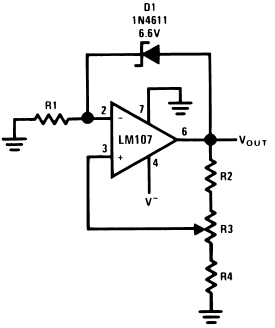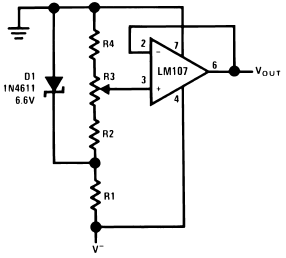### By internum

Adjustable voltage reference circuits (Positive and Negative).

Adjustable voltage reference circuits are shown in Figures 1, 2, 3, 4. The two circuits shown have different areas of applicability. The basic difference between the two is that Figures 1, 2 illustrate a voltage source which provides a voltage greater than the reference diode while Figures 3, 4 illustrates a voltage source which provides a voltage lower than the reference diode. The figures show both positive and negative voltage sources.Figure 1. Positive Voltage Reference

High precision extended temperature applications of the circuit of Figures 1, 2 require that the range of adjustment of VOUT be restricted. When this is done, R1 may be chosen to provide optimum zener current for minimum zener T.C. Since IZ is not a function of V+, reference T.C. will be independent of V+.Figure 2. Negative Voltage Reference

The circuit of Figures 3, 4 are suited for high precision extended temperature service if V+ is reasonably constant since IZ is dependent on V+. R1, R2, R3, and R4 are chosen to provide the proper IZ for minimum T.C. and to minimize errors due to Ibias.Figure 3. Positive Voltage Reference

The circuits shown should both be compensated for unity-gain operation or, if large capacitive loads are expected, should be overcompensated. Output noise may be reduced in both circuits by bypassing the amplifier input.Figure 4. Negative Voltage Reference

The circuits shown employ a single power supply, this requires that common mode range be considered in choosing an amplifier for these applications. If the common mode range requirements are in excess of the capability of the amplifier, two power supplies may be used. The LH101 may be used with a single power supply since the common mode range is from V+ to within approximately 2 volts of V−.221810 December 2007